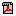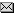Projecting Depreciation Rates for Machinery and BuildingsPDF(217K)Agri-NewsThis Week

On the Building Page (Bldg-Qta) and Machinery Page, there are cells to enter projected depreciation rates for the buildings and machinery shown on the statement. The rate chosen will bring those percentage of the values shown into the projections as an expense (the sum of the two shows as Depreciation in cell C38 on the DS SUM page). Obviously, the rate chosen, resulting in the amount of depreciation calculated, can have a big impact on projected profitability.
It is important to note, that depreciation is not the same as Capital Cost Allowance calculated on tax returns, so using the percentage rates that CRA allows are not necessarily appropriate. A definition of Depreciation that might provide clarification as it is used in this type of analysis is:

“the decrease in the value of an asset over time due to use, wear and tear, or obsolescence.”

You will notice if you look at those pages in ABA, that the projected ending values of those depreciable assets are the opening, or statement date values adjusted for the projected depreciation (with purchases and sales added in).

Given the above, the key to selecting a depreciation rate is estimating the amount that those assets will lose value over the upcoming year. If the assets are going to be retained, or considered to be assets until the end of their useful life, the depreciation rate would be the 1.00/# of years x 100 (assuming little or no salvage value at the end). If, for example, it is felt the remaining useful life of the line of equipment is 15 years, the depreciation rate would be 1.00/15x100 = 6.67%. If it is felt the useful life of the buildings on average is 40 years, the rate would be calculated to be 2.5%. As those are very rough estimates, it is likely appropriate, then, to round to the nearest decimal point. If equipment is going to be regularly replaced, then the depreciation amount would be the annual cost of replacing equipment. For example, if a new combine worth \$400,000 is going to be traded in at the end of 5 years, at which time the value will be \$250,000, the loss in value will be \$30,000/year, which would be approximately 7.5% in the upcoming year.

As can be seen, estimating projected depreciation, and depreciation rate is going to be very approximate, and will really vary on the expected life of the equipment, the repair and maintenance program being followed, and the replacement plan for depreciable assets. When the rate is selected, and the depreciation amount is calculated, a good check is to look at the depreciable asset replacement plan for the farm operation. The amount of depreciation for the projected year should be about equal to the dollars that would need to be spent annually, on average, to maintain a line of equipment at about the value shown in the statement. If, for example, the producer spends \$50,000 on equipment replacements to maintain the current line of equipment, that then should be about equal to the amount of projected depreciation in the ABA statement for the year.